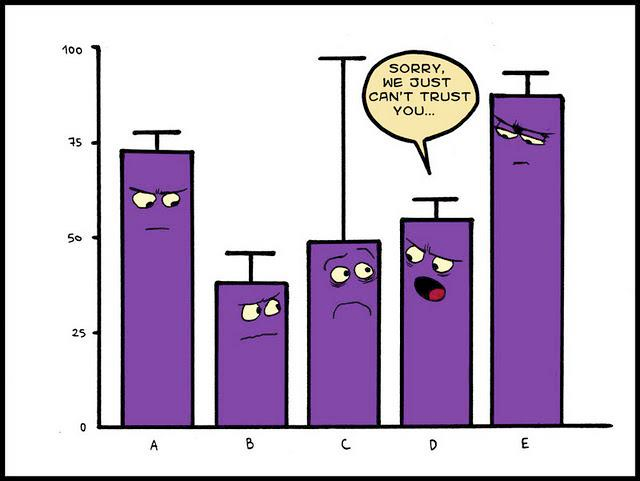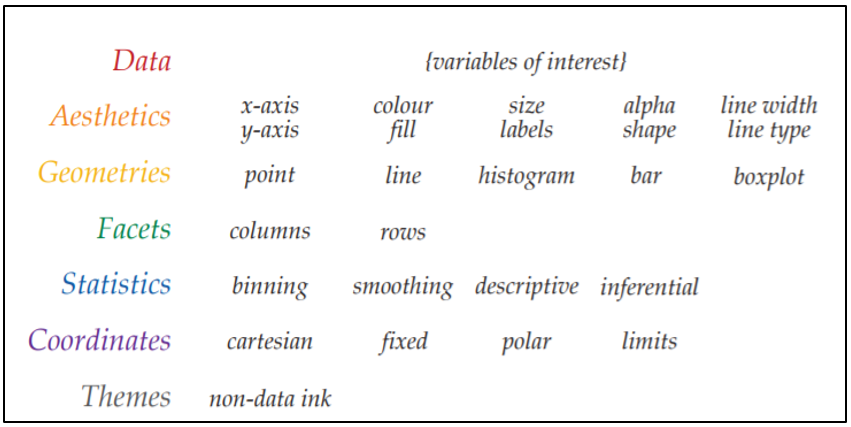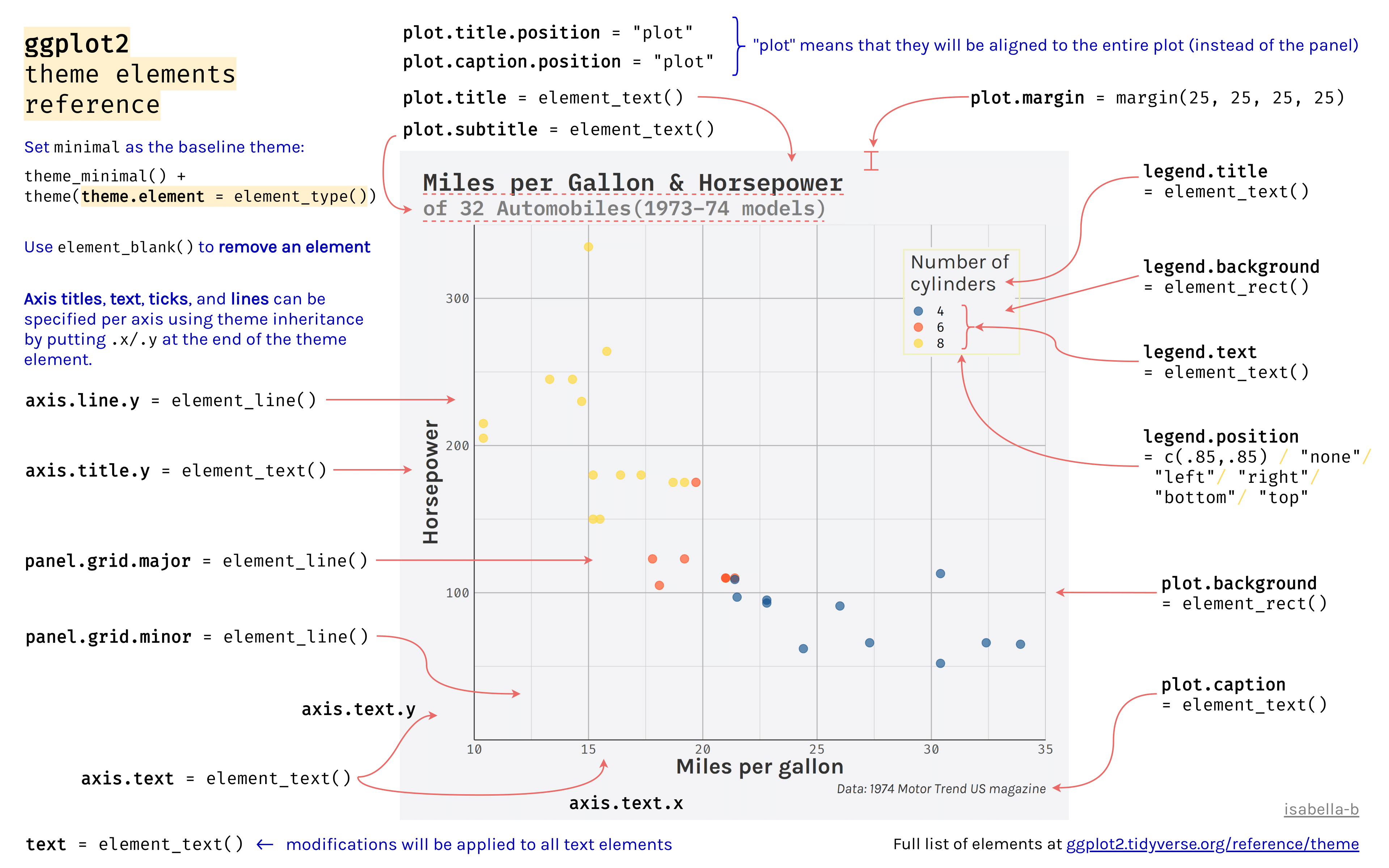# Chapter 10 Data VisualisationFigure 10.1: Why I use geom_point

`ggplot` allows you to create a variety of figures. These can be very simple or exceptionally complicated based on your desires. The important notion to keep in hand is that you can start with a simple figure, and then stack options over time.

I would highly recommend framing the ggplot cheat sheet until you get familiar with all the options at your disposal.

For those who are just beginning you may wish to use the `esquisse` package to help you along. Remember that GUIs as a whole are a crutch to lean on. As you get more comfortable I would highly recommend using code without the GUI.

For every figure we create we are going to save it in the `\images` directory within our project folder.

## 10.1 Anatomy of a ggplotFigure 10.2: Why I use geom_pointFigure 10.3: Why I use geom_point

## 10.2 For Beginners

For beginners this can all seem very very overwhelming. Please take your time learning this. I also would recommend using a few packages to help you get started. These GUIs are great to start, but know that they are limited. As you advance, learning the code will be a better option.

1. esquisse
2. ggthemeassist
3. ggedit

ggedit is similar to ggthemeassist you can read more about it here.

## 10.3 Looping with ggplot

In my work its common to want to create several figures based on a common column. In these instances we may want to “loop” through a variable creating several versions of a figure.

``````# Plots for 2 groups (OI, SRC) -----------
title_text <- paste0(res_05g2\$Conx[xx], " during ", res_05g2\$Task[xx], " (", res_05g2\$Result[xx], ")" )
x.text <- mriLocations\$Location[match(res_05g2\$location[xx], mriLocations\$Code)]
# if (res_05g2\$Component[xx] == "CohMean"){
#   y.text <- "Mean Coherence"
# }
# else if (res_05g2\$Component[xx] == "CohSTD"){
#   y.text <- "Variation of Coherence"
# }
#
plot1 <- ggplot(data = filter(df, Task == res_05g2\$Task[xx] & Connection == res_05g2\$Conx[xx] & a_group != "mTBI"),
aes(x=res_05g2\$location[xx], y=res_05g2\$Component[xx], color=a_group)) +
geom_jitter(size = 7) +
theme(legend.position = "top",
legend.title = element_text(size = 20),
legend.text = element_text(size = 20)) +
labs(x = x.text, y = res_05g2\$Component[xx], color = "Groups",
title = title_text)
tmp.txt <- str_replace(res_05g2\$Conx[xx],
"->" ," to ")
dir_lbl = paste0("images/test/", res_05g2\$location[xx],"_",res_05g2\$Component[xx], "_", res_05g2\$Task[xx],"_",tmp.txt ,".png") #using paste0 removes the spaces
ggsave(dir_lbl,plot1, width=11, height=8.5, dpi=400)
}``````

## 10.5 Resources for Drew

• Decent Presentation

• Go through each element in a ggplot. See here for scales

• Insert the paper comparing grammar of graphics vs MATLAB

• Add suggestion to join #tidytuesday which shows off just how fancy you can get. Its personally too much for my purposes and projects but it does give you an idea of what is possible. Try to notice the concepts that are being used. They are the same core principles that are shown here.

Why should you use ggplot? Well its based on the grammar of graphics which is an efficient way to plot your data. Here is a paper which demonstrates the efficiency of the grammar of graphics in MATLAB.

When inserting images into your RMarkdown document you can use the (Aspect Ratio Calculator] This chapter could be a book on its own, but we are going to go over a few different principles. First without many exceptions, we use `ggplot2` to create plots in R. There are a few other packages that depend on `ggplot2` which I use to model statistical data, but we will get to those at a later time.

For inspiration here are a couple of sites you can browse through

ggforce might be a good package to use for my 10-20 figuresFigure 10.4: My experience with MATLAB plots

You can find the ggplot cheatsheet here which you can refer to in case you are in need of inspiration.

You can also download the dataset we will be using from here

``````# Plotting -------------
journey_time\$year <- as.factor(journey_time\$year)

ggplot(data = df, aes(x= ..., y = ..., color = , ))

plot1 <- ggplot(journey_time) +
aes(x = service, fill = year, weight = journey_time_avg) +
geom_bar(position = "dodge") +
scale_fill_hue() +
labs(x = "Service", y = "Journey Time Average", title = "My Plot Name", fill = "Year") +
theme_minimal()

# Save the plot we created in "/images" folder ====================
ggsave("images/plot1.png",plot1, width=11, height=8.5, dpi=300)``````

If you find yourself using the same attributes over and over again you can save them in a list beforehand and then call them

`````` gglayers <- list(
geom_boxplot() ,
geom_point(size = 15, aes(shape = Gender, color = group2)) ,
scale_shape_manual(values=c("👧","👦"), name = "Sex") , # I need 9 values (I for each ID)
scale_color_manual(values=c('springgreen4', 'red4'), name = "Group", labels = c("Orthopedic-injured", "Brain-injured")) ,
scale_fill_manual(values =c('springgreen4', 'red4'), guide = F) ,
#facet_wrap(~hemisphere),
theme_minimal() ,
theme(legend.position = "top",
legend.title = element_text(size = 12),
plot.title = element_text(hjust = 0.5),
plot.caption = element_text(face = "italic"),
#legend.key = element_rect(colour = 'white', fill = 'white', size = 0.5, linetype='dashed'),
#legend.key.size = unit(2, "cm"),
#legend.key.width = unit(2, "cm"),
legend.text = element_text(size = 10)),
guides(shape = guide_legend(override.aes = list(size = 5)),
color = guide_legend(override.aes = list(size = 5)))
)

ggplot(data = df.hemi %>%
filter(mriloc2 == "CingC", metric == "MD")) +
aes(x = hemisphere, y = dtiChange) +
gglayers``````

Below is another example using a loop, which is a common task you might need to accomplish

``````# Making a loop to plot several items --------
# I have an example of this in JumpCut projects
# Example 1 =====================
#Now this will be for(i in 1:length(ERP_Component)) && each electNum
electName <- unique(erp\$electNum)
erpComp <- unique(erp\$ERP_component)
cond <- unique(erp\$Condition)
cond <- cond[sort.list(cond)]

for(ii in 1:(length(erpComp))) {
for(xx in 1:(length(electName))) {
tmp_erp <- subset(erp, ERP_component == erpComp[ii] & electNum == electName[xx])
#tmp_erp <- subset(erp, ERP_component == "P3b_Lat" & electNum == "E015")

#Now get each condition
lay = rbind(c(1,2), c(3,4)) #sets up the layout of my arranged plots
plot_list = list()
#p1 <- ggplot(data.frame(tmp2), aes(x=AthleteType), fill = AthleteType) + xlab("Athlete Type")

for(i in 1:(length(cond))) {
tmp2 <- subset(tmp_erp, Condition == cond[i]) #Creates a subset that has only GoC data when i=1
tmp3 <- tmp2 %>% group_by(Timet, AthleteType) %>%
summarise(mean=ci(ERP_value), lowCI=ci(ERP_value), hiCI=ci(ERP_value), sd=ci(ERP_value))
p1 <- ggplot(tmp3, aes_string(x=names(tmp3),y=names(tmp3),fill=names(tmp3)))  #dl = Group
p1 <- p1 + geom_bar(stat="identity") + geom_errorbar(aes(ymin=lowCI, ymax=hiCI),width=.2) + facet_wrap(~Timet) + scale_fill_grey() +
theme(legend.position="none", axis.text=element_text(size=8),axis.title.x=element_blank(), axis.title.y=element_blank(),plot.title = element_text(hjust = 0.5))
p1 <- p1 +
geom_jitter(data=tmp2,mapping= aes_string(x=names(tmp2), y = names(tmp2), color = names(tmp2)),position=position_jitter(width=.25, height=0),size = 2.9, alpha = 9/10) + theme(legend.position="none")

if (i==1){
p1 <- p1 + labs(title = "Correct/Go Condition")
} else if (i==2) {
p1 <- p1 + labs(title = "Correct/No-Go Condition")
} else if (i==3) {
p1 <- p1 + labs(title = "Incorrect/No-Go Condition")
}
plot_list[[i]] <- p1
}
# Let's create our y axis label
tmp_lbl <- unique(tmp2\$ERP_component)
underscoreLocation <- unlist(gregexpr(pattern ='_',tmp_lbl)) #Gather the location of the underscore needed to create the labels

if (endsWith(tmp_lbl,"Lat") ==1){
#Here I need to get the ERP Component Name. I had this in a loop but because of the different sizes (N2 = 2 vs ERN = 3 characters)
tmp_CompName = substr(tmp_lbl,start=1,stop=underscoreLocation-1)
tmp_lbl2 <- paste(electName[i],tmp_CompName,"Local Peak Latency (ms)")
p <- grid.arrange(plot_list[],plot_list[],plot_list[], layout_matrix = lay,
bottom = textGrob("Note: Different colors in the jitter represent different athletes",
gp=gpar(fontsize=12,font=3), hjust=0.1),
left = textGrob(paste(tmp_lbl2), rot = 90, vjust = 1))
#p
dir_lbl = paste0("images/ERP_GroupBar/PeakLat/",tmp_CompName,"PeakLat_",unique(tmp2\$electNum),".png") #using paste0 removes the spaces
ggsave(dir_lbl,p, width=11, height=8.5, dpi=400)

}else if (endsWith(tmp_lbl,"Amp") ==1){
tmp_CompName = substr(tmp_lbl,start=1,stop=underscoreLocation-1)
tmp_lbl2 <- paste(tmp_CompName,"Local Peak Amplitude (")

p <- grid.arrange(plot_list[],plot_list[],plot_list[], layout_matrix = lay,
bottom = textGrob("Note: Different colors in the jitter represent different athletes",
gp=gpar(fontsize=12,font=3), hjust=0.1),
left = textGrob(bquote(.(tmp_lbl2)*mu*.("V)")), rot = 90, vjust = 1))
#p
dir_lbl = paste0("images/ERP_GroupBar/PeakAmp/",tmp_CompName,"PeakAmp_",unique(tmp2\$electNum),".png") #using paste0 removes the spaces
ggsave(dir_lbl,p, width=11, height=8.5, dpi=400)
}
}
}
rm(underscoreLocation, tmp_CompName, tmp_lbl,tmp_lbl2, dir_lbl, i, ii, xx, electName, erpComp, cond, tmp3, tmp2, lay)

# Example 2 ==============
#Create a string with the y-labels
out <- NULL
p <- ggplot(data.frame(dl), aes(x=dl\$Groupt), fill = Groupt) + xlab("Groups")
for(i in 10:(ncol(dl)-0)) {
p <- ggplot(dl, aes_string(x=names(dl), y = names(dl)[i], fill = names(dl)))
p <- p + stat_summary(fun.y = mean, geom = "bar") + stat_summary(fun.data = mean_cl_normal, geom = "errorbar", width = 0.2) + facet_wrap(~CondBt) + theme(legend.position="none", axis.title.x=element_blank())
print(p)
out[[i-9]] <- p
}
s1 <- marrangeGrob(grobs=out, nrow = 2, ncol=2)
ggsave("try2.pdf",s1)
dev.off``````

## 10.6 Activity 1: Merging Figures

In a recent project, I found myself with the task to merge images I had created for a manuscript. The image would be in a 2x2 grid with labels A,B,C and D. In most circumstances, the images would be created in ggplot, but in this case one of them was a `.png` file which meant I could not use `ggarrange`.Example of figure setup

Over a day of work later, I looked at a series of packages to try and acheive my goal. Here are the list of programs I looked into on my adventure.

1. imager `imager` looked like a promising option. However, I was never able to get the images to grid properly. I posted an issue on GitHub but have yet to hear back. I don’t think it will do what we need here.

2. cowplot

`cowplot` was a great contender. I used the code found on their vignette here

From their own vignettes, `.png` files can only be used as backgrounds. I don’t see an option to include an imported image within the plot list. An example is shown below. `ggarrange` is best if you have ggplot figures because it will resize for you.

``````mypngfile <- download.file("https://upload.wikimedia.org/wikipedia/commons/thumb/e/e4/France_Flag_Map.svg/612px-France_Flag_Map.svg.png",
destfile = "france.png", mode = 'wb')
I would highly recommend the presentation found in `misc/presentation.pdf`